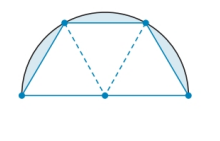Chapter 8.4, Problem 46E### Elementary Geometry for College St...

6th Edition
Daniel C. Alexander + 1 other
ISBN: 9781285195698

#### Solutions

Chapter
Section### Elementary Geometry for College St...

6th Edition
Daniel C. Alexander + 1 other
ISBN: 9781285195698
Textbook Problem
1 views

# A tabletop is semicircular when its three congruent drop-leaves are used. By how much has the tables area increased when the drop leaves increased. Give the answer to the nearest whole percent.To determine

To Find:

The percentage of increased area of the tabletop.

Explanation

The tabletop contains three congruent triangles.

The side of the triangle is equal to the radius of the circle.

Therefore, s=r.

The area of the equilateral triangle is A=3s24=3r24.

Therefore, the area of the three triangles is A=33r24.

The area of the semicircle is A=12πr2.

To find the area of the drop-leaves of the tabletop, subtract the area of three triangles from the area of the semicircle

### Still sussing out bartleby?

Check out a sample textbook solution.

See a sample solution

#### The Solution to Your Study Problems

Bartleby provides explanations to thousands of textbook problems written by our experts, many with advanced degrees!

Get Started

#### Construct an angle that measures 75.

Elementary Geometry For College Students, 7e

#### x234x5

Applied Calculus for the Managerial, Life, and Social Sciences: A Brief Approach

#### Differentiate the function. F(s) = ln ln s

Single Variable Calculus: Early Transcendentals, Volume I

#### The range of f(x) = tan1 x is: a) [2,2] b) (2,2) c) (0,2) d) all reals

Study Guide for Stewart's Single Variable Calculus: Early Transcendentals, 8th

#### The gradient vector field for is:

Study Guide for Stewart's Multivariable Calculus, 8th

#### Rationalize each denominator. 75

College Algebra (MindTap Course List)